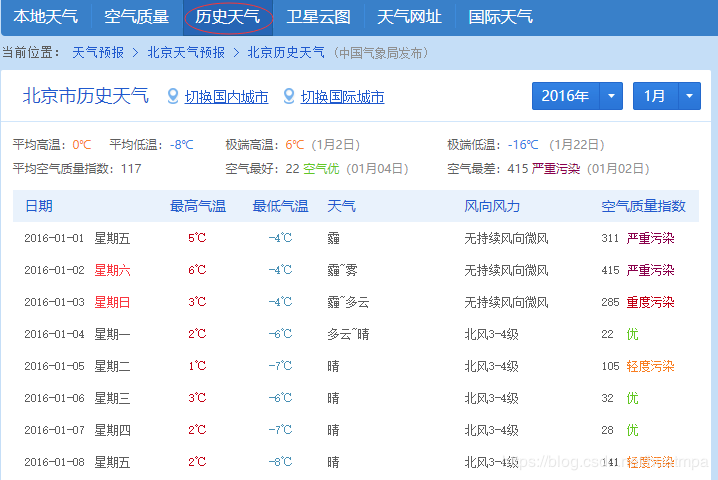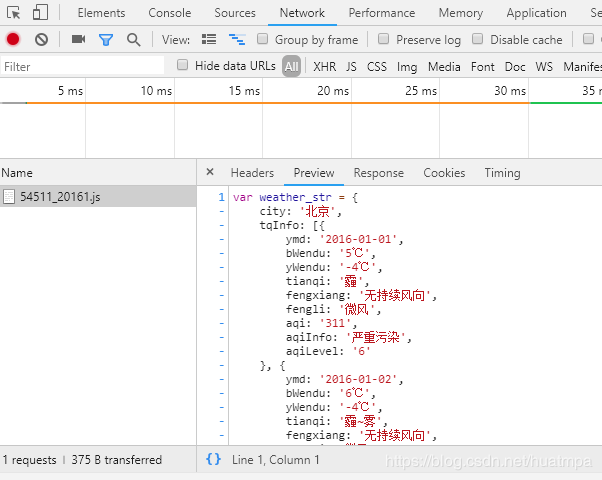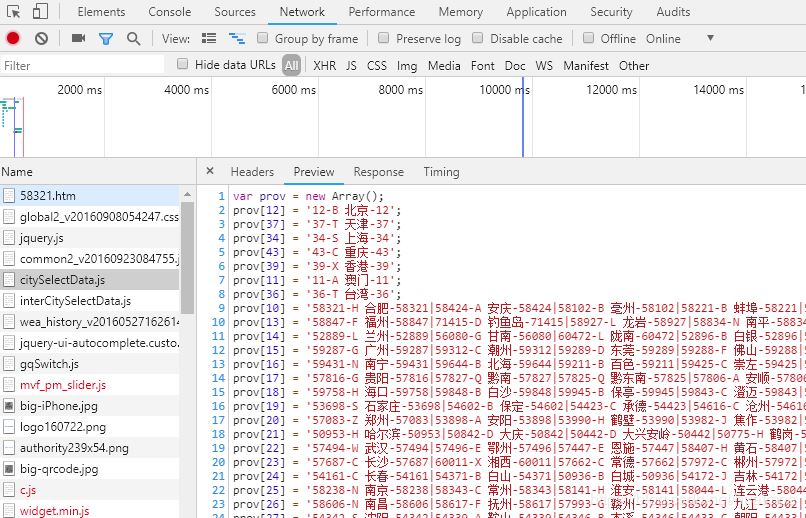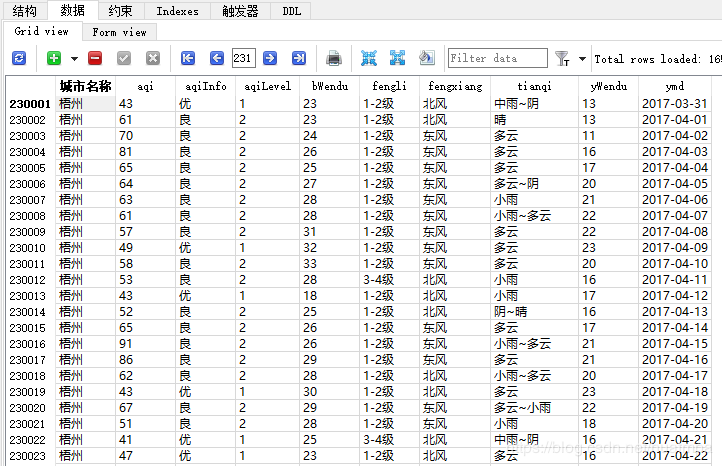# 用python爬取历史天气数据的方法示例

yipeiwu_com3年前Python爬虫url中的“54511”是城市代码，“20161”是年份和月份代码。下一步就是找到城市代码列表，按城市+年份+月份构造url列表，就能开始遍历爬取了。```def getCity():
html = reqs.get('https://tianqi.2345.com/js/citySelectData.js').content
text = html.decode('gbk')
city = re.findall('([1-5]\d{4})\-[A-Z]\s(.*?)\-\d{5}',text)  #只提取了地级市及以上城市的名称和代码，5以上的是县级市
city = list(set(city))                    #去掉重复城市数据
print('城市列表获取成功')
return city
```

```def getUrls(cityCode):
urls = []
for year in range(2011,2020):
if year <= 2016:
for month in range(1, 13):
urls.append('https://tianqi.数字.com/t/wea_history/js/%s_%s%s.js' % (cityCode,year, month))
else:
for month in range(1,13):
if month<10:
urls.append('https://tianqi.数字.com/t/wea_history/js/%s0%s/%s_%s0%s.js' %(year,month,cityCode,year,month))
else:
urls.append('https://tianqi.数字.com/t/wea_history/js/%s%s/%s_%s%s.js' %(year,month,cityCode,year,month))
return urls
```

```def getHtml(url):
header = {'User-Agent': 'Mozilla/5.0 (Windows NT 6.1; WOW64; rv:14.0) Gecko/20100101 Firefox/14.0.1',
'Referer': '******'}
text = request.content.decode('gbk') #经试解析，这里得用gbk模式
time.sleep(random.randint(1,3))    #随机暂停，减轻服务器压力
return text
```

2016年开始的数据和之前年份不一样，多了PM2.5污染物情况，因此构造正则表达式时，还不能用偷懒模式。

```str1 = "{ymd:'(.*?)',bWendu:'(.*?)℃',yWendu:'(.*?)℃',tianqi:'(.*?)',fengxiang:'(.*?)',fengli:'(.*?)',aqi:'(.*?)',aqiInfo:'(.*?)',aqiLevel:'(.*?)'.*?}"
str2 = "{ymd:'(.*?)',bWendu:'(.*?)℃',yWendu:'(.*?)℃',tianqi:'(.*?)',fengxiang:'(.*?)',fengli:'(.*?)'.*?}"

#这个就是偷懒模式，取出来的内容直接存入元组中```

```def getDf(url):
html = getHtml(url)
pa = re.compile(r'{(ymd.+?)}')           #用'{ymd'打头，把不是每日天气的其它数据忽略掉
text = re.findall(pa,html)
list0 = []
for item in text:
s = item.split(',')              #分割成每日数据
d = [i.split(':') for i in s]         #提取冒号前后的数据名称和数据值
t = {k:v.strip("'").strip('℃') for k,v in d} #用数据名称和数据值构造字典
list0.append(t)
df = pd.DataFrame(list0)              #加入pandas列表中，便于保存
return df ```

```def work(city,url):
con =sql.connect('d:\\天气.db')
try:
df = getDf(url)
df.insert(0,'城市名称',city)                #新增一列城市名称
df.to_sql('total', con, if_exists='append', index=False)
print(url,'下载完成')
except Exception as e:
print("出现错误:\n",e)
finally:
con.commit()
con.close()```